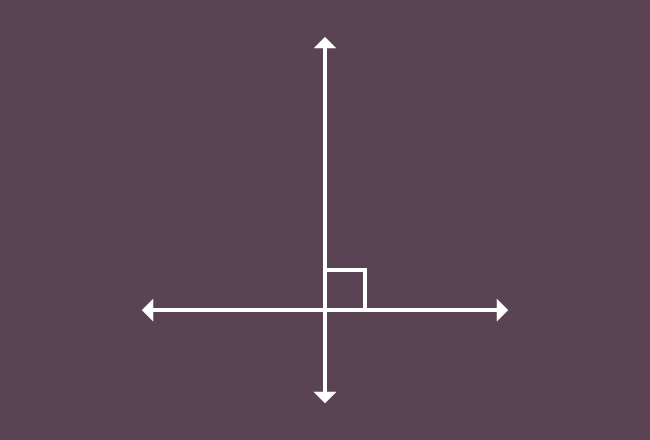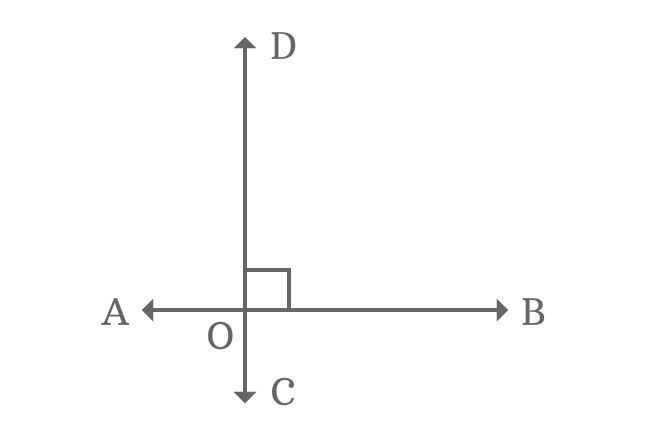# Perpendicular LinesThe intersecting lines which contain right angle between them are called perpendicular lines.

## Introduction

Straight lines are often intersected in a plane in geometrical system. Occasionally, they get intersected by an angle of $90$ degrees (right angle).

In this case, the straight lines are called as perpendicular lines.

### Example

$\small \overleftrightarrow{AB}$ and $\small \overleftrightarrow{CD}$ are two straight lines and they are intersected by an angle of $90^\circ$ in a plane at point $\small O$. Therefore, the straight lines $\small \overleftrightarrow{AB}$ and $\small \overleftrightarrow{CD}$ are said to be perpendicular to each other.1. $\small \overleftrightarrow{AB}$ is called as perpendicular line to $\small \overleftrightarrow{CD}$, and represented by $\small \overleftrightarrow{AB} \perp \overleftrightarrow{CD}$.
2. $\small \overleftrightarrow{CD}$ is called as perpendicular line to $\small \overleftrightarrow{AB}$, and denoted by $\small \overleftrightarrow{CD} \perp \overleftrightarrow{AB}$.
Latest Math Topics
Jun 26, 2023
Jun 23, 2023

Latest Math Problems
Jul 01, 2023
Jun 25, 2023
###### Math Questions

The math problems with solutions to learn how to solve a problem.

Learn solutions

Practice now

###### Math Videos

The math videos tutorials with visual graphics to learn every concept.

Watch now

###### Subscribe us

Get the latest math updates from the Math Doubts by subscribing us.